### Home > INT3 > Chapter 11 > Lesson 11.2.2 > Problem11-70

11-70.
1. Simplify each expression. Homework Help ✎

2.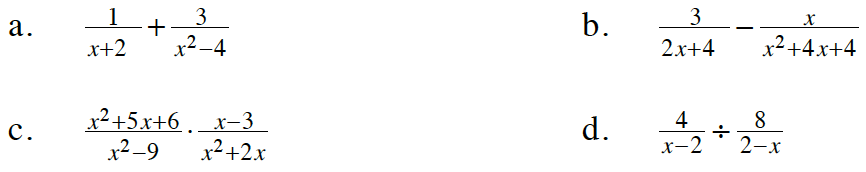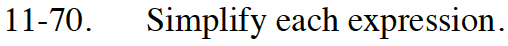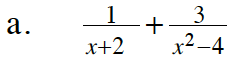Begin by factoring x2 − 4.

Multiply the first term by a Giant One that will make the two denominators the same.

$\frac{x-2}{x -2} \cdot \frac{1}{x+2} + \frac{3}{(x-2)(x+2)}$

$\frac{x+1}{(x+2)(x-2)}$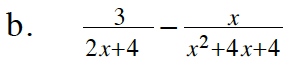Factor both denominators.

Refer to part (a).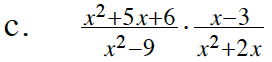Factor all parts of the problem that can be factored. Remove Giant Ones.

$\frac{1}{x}$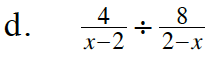Remove Giant Ones.

$\text{What's }\frac{2-x}{x-2}?$

If you aren't sure, substitute different numbers for x.

$\frac{4}{x-2} \cdot \frac{2-x}{8}$

$\frac{4}{8} \cdot \frac{2-x}{x-2}$

$\frac{1}{2}\cdot -1$